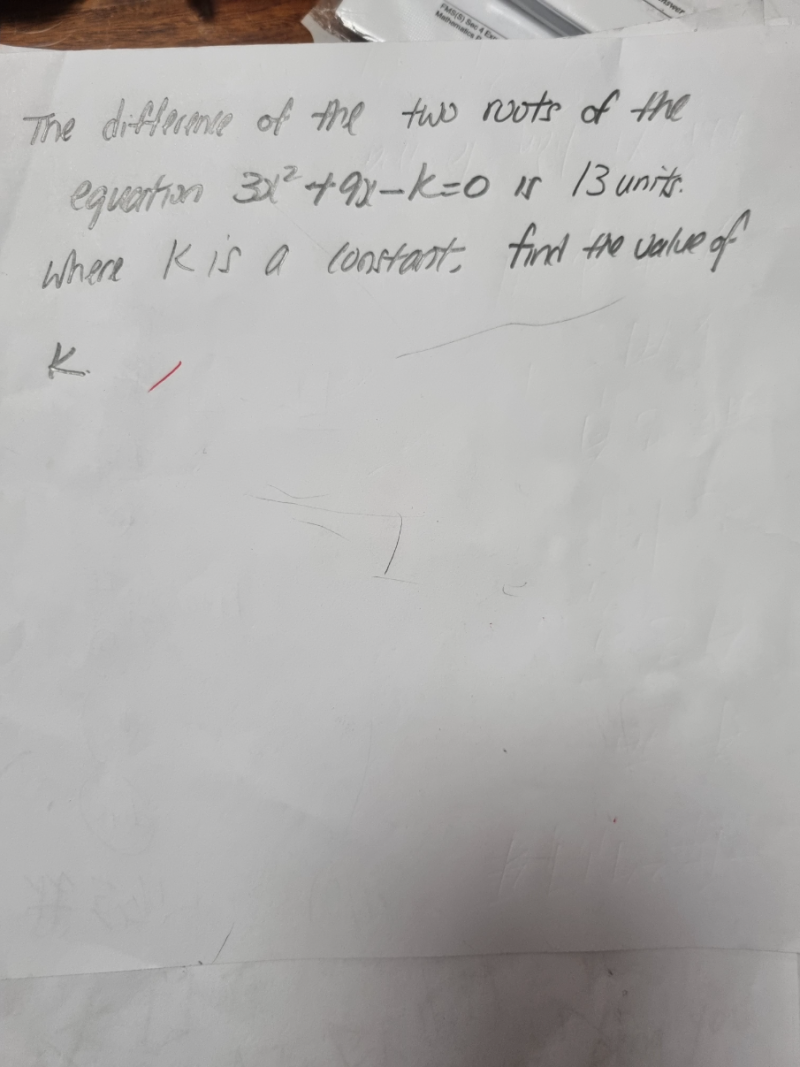# QuestionThank you.

3x2 + 9x – k = 0       has a root difference of 13 units.

=>  x23x – (k/3) = 0

Let the roots be a and b.

(x-a)(x-b) = 0   =>  x2 – (a+b)x + ab = 0

– (a + b) =  =>   a + b = -3 ————(1)

It is given that  a- b = 13 —————-(2)

ab = – (k/3) ——————————-(3)

(1) + (2),

2a = 10 => a = 5

b = -3 -5 = -8

Sub, a=5 and b = -8 into (3)

-(k/3) = (5)(-8) = -40

k = 40 x 3 = 120

0 Replies 1 Like